# Compartment model

Compartment models can be used to model the transport processes between interconnected volumes, such as the flow of drugs and hormones in the human body (right). Compartment models assume that there is perfect mixing so that the drug concentration is constant in each compartment. The complex transport processes are approximated by assuming that the flow rates between the compartments are proportional to the concentration differences in the compartments. One of the early uses of compartment modes was by Widmark in the 1920s, who modeled the propagation of alcohol in the body. Compartment models are now important for the screening of all drugs used by humans.

## Two compartment model

A simple two-compartment model is shown to the right. We assume that there is perfect mixing in each compartment and that the transport between the compartments is driven by concentration differences. We further assume that a drug with concentrationis injected in compartment 1 at a volume flow rate ofand that the concentration in compartment 2 is the output. Let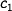and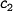be the concentrations of the drug in the compartments and letand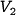be the volumes of the compartments.

The dynamics of the system can be obtained by keeping track of the flow rates into and out of each compartment. The mass balances for the compartments arewhererepresents flow rate between the compartments and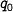represents the flow rate out of compartment 1 that is not going to compartment 2. Introducing the variables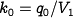,,andand using matrix notation, the model can be written as